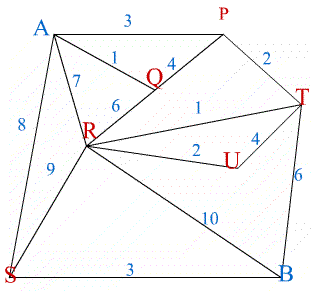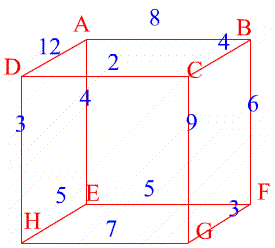#### You may also like### Instant Insanity

Given the nets of 4 cubes with the faces coloured in 4 colours, build a tower so that on each vertical wall no colour is repeated, that is all 4 colours appear.### Tree Graphs

A connected graph is a graph in which we can get from any vertex to any other by travelling along the edges. A tree is a connected graph with no closed circuits (or loops. Prove that every tree has exactly one more vertex than it has edges.### Magic Caterpillars

Label the joints and legs of these graph theory caterpillars so that the vertex sums are all equal.

# Maximum Flow

##### Age 16 to 18Challenge LevelThe graph represents a supply network from $A$ to $B$ and the numbers on the edges of the graph show the maximum capacity for flow in each of the sections.

Imagine any straight line cutting through edges of the graph (but not through vertices) such that $A$ is on one side of the line and $B$ is on the other. All the flow from $A$ to $B$ has to go along the edges cut by your line so the total flow from $A$ to $B$ is less than or equal to the sum of the flows along those edges. Considering all possible such cuts, why is it that the maximum flow from $A$ to $B$ is less than the minimum sum for all cuts? Find the maximum flow in this example.In the second example the network is a cube. Find the maximum flow from $A$ to $G$.